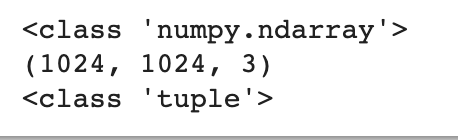# About my poor performance in anomaly detection

I trained an autoencoder for screw image anomaly detection, the model is like:
class Autoencoder(nn.Module):
def init(self):
super(Autoencoder, self).init()
self.encoder = nn.Sequential(
nn.Linear(256, 128),
nn.ReLU(True),
nn.Linear(128, 64),
nn.ReLU(True),
nn.Linear(64, 32))

``````    self.decoder = nn.Sequential(
nn.Linear(32, 64),
nn.ReLU(True),
nn.Linear(64, 128),
nn.ReLU(True),
nn.Linear(128, 256),
nn.Tanh())

def forward(self, x):
y = self.encoder(x)
y = self.decoder(y)
return y
``````

the training loss and valid loss went well
and the threshold is set in this way.
but it gave a really poor outcome, anyone can give me a hint?

What is the size of your images?

that’s the transform and I reshape it to 1 dimension when I sent it to train

And what is your original image size before resize?I think is 102410243

This is quite a resize. May🐝 you do like this:

``````nn.Linear(1024*1024*3, 1024),
nn.ReLU(True),
nn.Linear(1024, 64),
nn.ReLU(True),
nn.Linear(64, 32))
``````

and create a symmetrical decoder. What is your specific task of anomaly detection. Noise reduction?

I’ll let you know how it goes after I try it.It turns slightly better after I modified the net size.

Can you share the training block of your code? You might have some bugs there. In addition, it seems that you are using default weight values provided by `PyTorch`, which isn’t ideal, check this for more info. It is better to initialize your layer weights with a better method, such as `Xavier`, to ensure the convergence and the stability of training, check this Stackoverflow answer for more information.

As you are dealing with images, why are you collapsing the image dim into a vector? Here is an example to show what you are doing:

``````import copy

import numpy as np
from PIL import Image
import torch
import torchvision
import torchvision.transforms as T

x = np.random.rand(1024, 1024, 3)
img = Image.fromarray(np.uint8(x))
print(x.shape) # (1024, 1024, 3)
print(img.size) # (1024, 1024)

transform = T.Compose([
torchvision.transforms.Resize((16,16)),
T.ToTensor(),
])

imgs = torch.stack([copy.deepcopy(transform(img)) for i in range(10)])
print(imgs.shape) # torch.Size([10, 3, 16, 16])

imgs_ = imgs.view(imgs.size(0), -1)
print(imgs_.shape) # torch.Size([10, 768])
``````

What are your loss, optimizer, and hyperparameters?

In addition, why do you need to normalize your image to be with the mean and the std of `0.5`? We usually normalize based on the dataset mean and std not setting up a mid-point value. This might suppress information.

In the future, please share code snippets rather than screenshots for code so we can test things.

Edit: noticed a bug in the above code

I coded in Colab so I can’t subtly handle the datasets due to the limited RAM.

The hyperparameter: criterion = nn.MSELoss() and the SGD optimizer with lr = 1e-3, momentum = 0.9
I also added scheduler back then to check the loss curve.

I believe your main issue is using an MLP to detect anomaly on images. It is better to have a feature extractor, ConvNet layers, then your MLP. In addition, we typically resize the images using `torchvision.transforms.Resize` to a lower size (e.g. (244,244)) to fit into GPU memory.

Having a feature extractor then an MLP means your MLP will have well-encoded and rich information coming from the feature extractor and the MLP will have an easier time finding pattern between the features for your desired task.

Thanks your tips. I just inspired by the tutorial of anomaly detection for mnist. And it was build in tensorflow and its network consists of multiple linear units. So I did an impression based on it and it did not go well sadly.

Sometime the free GPU ran out so I have to switched the accelerator between of them.

MNIST is a pretty simple dataset as you have small, grayscale, and easy to classify images. However, for large, colored, and hard images, you will need a better model to learn the right features to solve the task at hand. It isn’t about using TensorFlow or PyTorch. It is about not solving the problem in the right way.